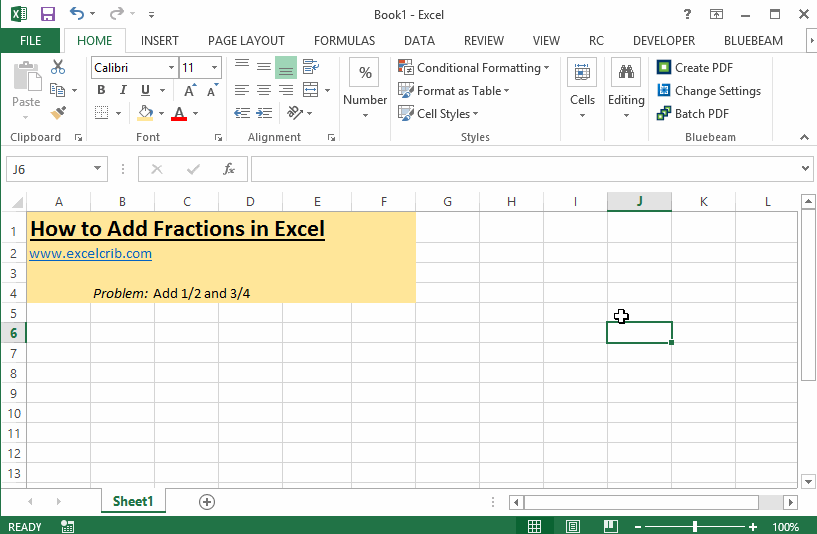# How to Add Fractions in Excel

In the previous tutorial, we learned how to add in Microsoft Excel, but do you know that it is also capable of solving fraction problems. This tutorial will show how to add fractions.

## Steps

1. Input the fractions as a regular formula in individual cells. Excel will automatically convert it to decimal format. For example =1/2 will yield 0.5 in a cell.
2. Add the numbers using cell reference separated by + symbol. Again Excel will convert it into a decimal formal.
3. Select the cells where you want to convert to fraction format, then format cells (can be accessed by either right-clicking or from the ribbon menu). A small window will pop out, then select 'Number' tab, choose 'Fraction' and press 'OK'.

Watch the screen recording below for demonstration.You might also want to learn how to divide fractions, see our next tutorial.

Excelcrib was founded in November 2017 by Garry, a Microsoft® Office Excel® (MS Excel) enthusiast with background in engineering. He's been using MS Excel for more than 15 years in practice with specialty in VBA.